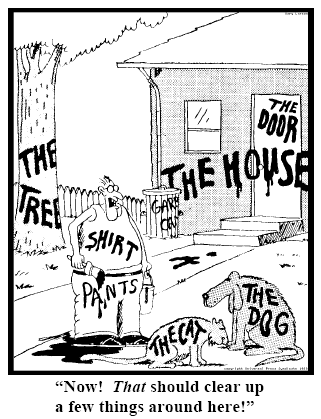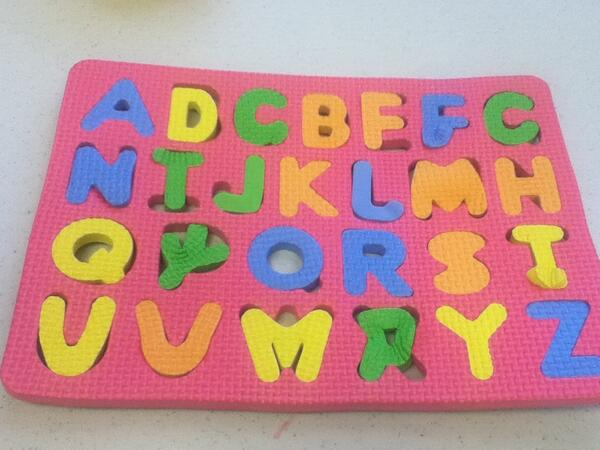# Elm: Your next front end

Igor Kapkov

## Types!!!111## Example

``````> import String
> String.repeat
<function: repeat> : Int -> String -> String```
```> myWrap a = [a]
<function> : a -> List a```
`> String.repeat 2 (myWrap 1)````

## Result

`TypeError: 'undefined' is not a function`## Result

``````-- TYPE MISMATCH
The 2nd argument to function `repeat` is causing a mismatch.
4│   String.repeat 2 (myWrap 1)
^^^^^^^^
Function `repeat` is expecting the 2nd argument to be:
String
But it is:
List number

Hint: I always figure out the type of arguments from left to right. If an
argument is acceptable when I check it, I assume it is "correct" in subsequent
checks. So the problem may actually be in how previous arguments interact with
the 2nd.``````

## More

````type User = Anonymous | LoggedIn String`
`type Maybe a = Just a | Nothing`
`type List a = Empty | Node a (List a)`
`type Tree a = Empty | Node a (Tree a) (Tree a)````

## Extensible Records

````{ x = 3, y = 4 }`
```type alias Person = { first_name : String
, last_name : String
}```
`author = Person "Igor" "Kapkov"`
`author.first_name`
`{ author | first_name = "Igas" }`
Extensible records with scoped labels by Daan Leijen
```

## FRP

``````> a = 1
1 : number```
```> b = a + 1
2 : number```
```> a = 2
2 : number```
```> b
3 : number``````

## FRP example #1

``````import Graphics.Element exposing (..)
import Mouse
main : Signal Element
main =
Signal.map show Mouse.position``````

## FRP example #2

``````import Graphics.Element exposing (..)
import Mouse
main : Signal Element
main =
Signal.map show countClick
countClick : Signal Int
countClick =
Signal.foldp (\clk count -> count + 1) 0 Mouse.clicks``````

## FRP example #3

``````import Graphics.Element exposing (..)
import Mouse
main : Signal Element
main =
Signal.map show lastPositions```
```lastPositions : Signal (List (Int, Int))
lastPositions =
Signal.map (List.take 3) allPositions``````

## FRP example #3

``````allPositions : Signal (List (Int, Int))
allPositions =
Signal.foldp
(\clk count -> clk :: count)
[]
positionAfterClick```
```positionAfterClick : Signal (Int, Int)
positionAfterClick =
Signal.sampleOn Mouse.clicks Mouse.position``````

• model
• update
• view

## Elm.Html

``````import Html as T

main =
T.ol
[ ]
[ T.li [] [ T.text "hey" ]
, T.li [] [ T.text "ho" ]
]``````

## the Elm Architecture

``````module CounterApp where

import Counter exposing (update, view)
import StartApp.Simple exposing (start)

main =
start
{ model = 0
, update = update
, view = view
}``````

## the Elm Architecture

``````module Counter where

import Html exposing (..)
import Html.Attributes exposing (style)
import Html.Events exposing (onClick)

-- MODEL

type alias Model = Int``````

## the Elm Architecture

``````-- UPDATE

type Action = Increment | Decrement

update : Action -> Model -> Model
update action model =
case action of
Increment ->
model + 1

Decrement ->
model - 1``````

## the Elm Architecture

``````-- VIEW

view : Signal.Address Action -> Model -> Html
div []
[ button [ onClick address Decrement ] [ text "-" ]
, span [ ] [ text (toString model) ]
, button [ onClick address Increment ] [ text "+" ]
]``````

## True SemVer

``````\$ elm package diff evancz/elm-html 1.0.0 1.1.0
Comparing evancz/elm-html 1.0.0 to 1.1.0...
This is a MINOR change.
------ Changes to module Html.Attributes - MINOR ------
classList : List (String, Bool) -> Attribute``````

## True SemVer

``````\$ elm package diff evancz/elm-html 1.1.0 2.0.0
Comparing evancz/elm-html 1.1.0 to 2.0.0...
This is a MAJOR change.
------ Changes to module Html.Attributes - MAJOR ------
attribute : String -> String -> Attribute
minlength : Int -> Attribute
Changed:
- colspan : String -> Attribute
+ colspan : Int -> Attribute
- rowspan : String -> Attribute
+ rowspan : Int -> Attribute``````